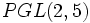# PGL(2,5) is isomorphic to S5

This article gives a proof/explanation of the equivalence of multiple definitions for the term symmetric group:S5
View a complete list of pages giving proofs of equivalence of definitions

## Statement

The projective general linear group of degree two over field:F5, denoted$PGL(2,5)$, is isomorphic to symmetric group:S5.

## Facts used

1. PSL(2,5) is isomorphic to A5
2.$S_5$ is the automorphism group of$A_5$
3.$PGL(2,p)$ is the automorphism group of$PSL(2,p)$ for any prime$p$

## Proof

The proof follows directly by combining Facts (1)-(3).